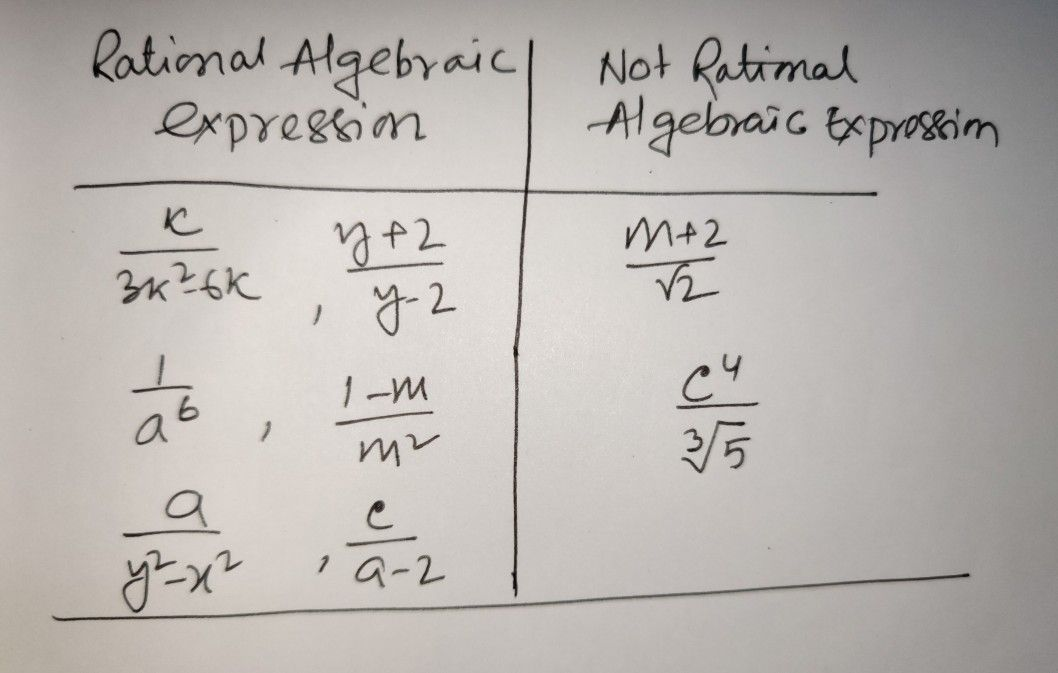Symbol
ProblemMathematics Quarter $1$ Week $3$ $AcinH$ Sheet $Name\right)$ $5ecii0n$ $Acbnx$ $13$ Classify the given expressions as Rational Expression or Not Rational Algebraic Expression, then answer the following questions in your answer sheets. Rational Algebraic Not Rational Algebraic $0$ $\dfrac {m+2} {\sqrt{2} }$ $\dfrac {k} {3k^{2-6k\right)}}$ Expression Expression $\dfrac {1} {a^{0}}$ $0$ $\dfrac {y+2} {y-2}$ $\dfrac {1-m} {m^{3}}$ $\dfrac {c} {a-2}$ $\dfrac {c} {\sqrt{5} }$ $\dfrac {a} {y^{2-x\right)}}$
7th-9th grade
Algebra
Search count: 105
Question content
pls help me about this!!
SolutionQanda teacher - RaiKindly Evaluate the Answer ?? if you're satisfied.Student
thank you so much?
I thought no one can solve thisQanda teacher - Rai
my pleasure
now please Evaluate the Answer ?Student
ma'am me I ask u?
can u do it again clearly?